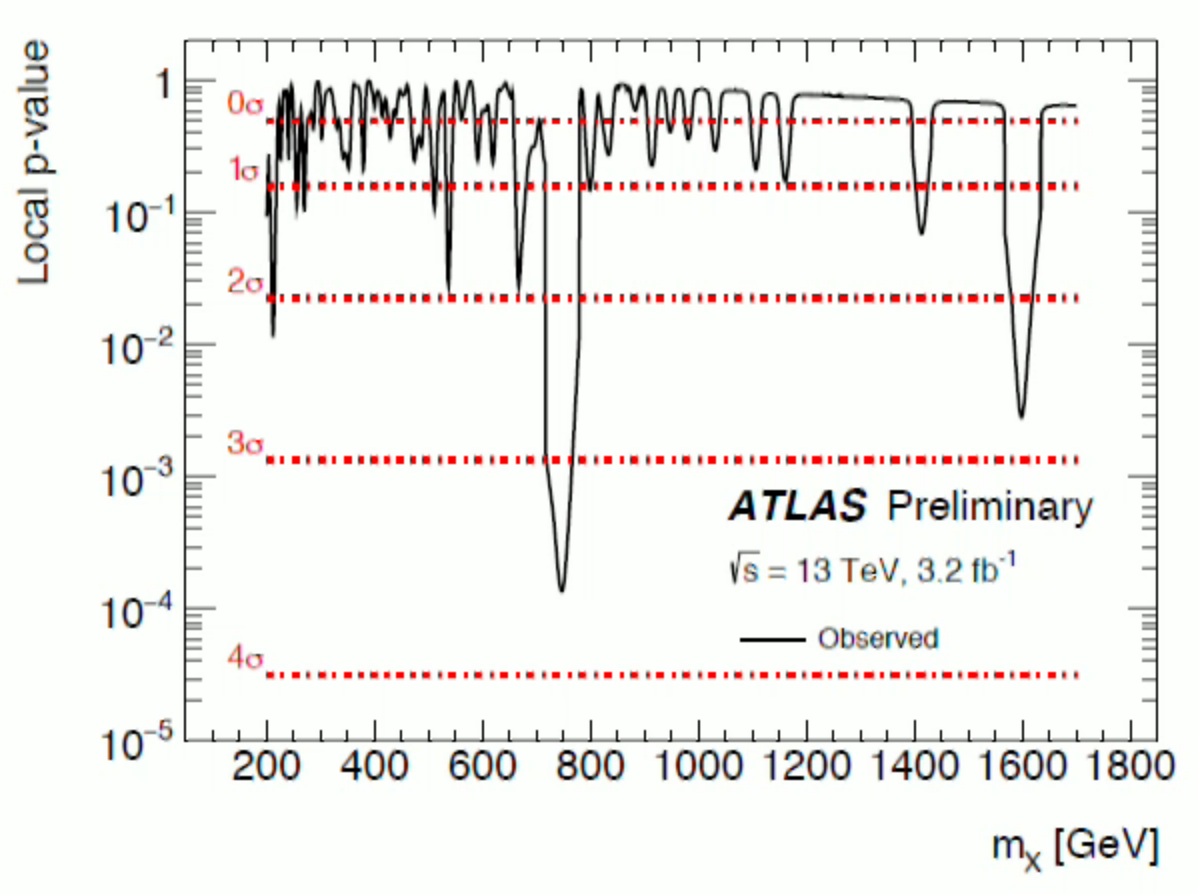## Wednesday, March 23, 2016 ...//### LHC: some new weak deviations from the Standard Model

ATLAS and CMS have released lots of papers in recent days. I will very briefly mention a few ATLAS graphs you may find interesting.

In a search for $$ZZ\to\ell\ell qq$$ resonances, Figure 4 shows a 2-sigma (locally) spread excess for $$m_H\sim 950\GeV$$. Between $$450\GeV$$ and $$750\GeV$$, there is a comparably strong deficit.

In a search for a CP-odd Higgs decaying as $$A\to Zh\to Zb \bar b$$, you may see a 2-sigma excess on Figure 8 for $$m_A \sim 500\GeV$$ and perhaps $$m_A\sim 280\GeV$$, too.

When they searched for heavy boosted top quarks, they saw signs of a more than 2-sigma deficit for the mass $$m_{Z'}\sim 3\TeV$$. Ironically, the deficit appears close to the mass of a $$Z'$$ boson where we used to have an excess sometime in the past, just half a year ago.

Those weren't spectacular deviations. The following one isn't, either, but it seems stronger and may have a better story. In the search for Higgs pairs decaying as $$hh\to b\bar b b \bar b$$, Figure 10b shows something that looks almost like a 3-sigma excess centered at $$m_{G^*} \sim 1,650\GeV$$. The main text only discusses the smaller deviations at similar graphs on Figures 10a, 10c.

Figure 10b is interpreted as a sign of a heavy Randall-Sundrum massive graviton with $$k / \tilde M_{Pl} =2$$, a basic parameter of the warped geometry scenarios. This is a value for which you get a stronger excess – and the excess is stronger with the Randall-Sundrum spin-two interpretation than if you assume it is a scalar. What seems interesting about this value is that ATLAS has also seen a nearly 3-sigma diphoton excess near the invariant mass of $$1.6\TeV$$ – in the very same search that made us excited about the cernette excess at $$750\GeV$$ in December 2015.Most of similarly strong hints go away in the history of particle physics. It's always plausible that some of them won't. I do think that the probability that a new $$750\GeV$$ elementary particle exists is comparable to 50%. The probability for a new $$1.6\TeV$$ particle is smaller, say 5%, but it's plausible that in the future, we will be able to look at these very weak hints as the "emerging evidence" we will have could (correct the tense) pay more attention to.

There also exists an interesting theoretical proposal that aside from the $$750\GeV$$ new particle $$\phi_1$$, there should also exist a particle $$\phi_2$$ of a "slightly more than double" mass, $$1.6\TeV$$, which has the advantage that the heavier particle decays to a pair of the lighter ones $$\phi_2\to \phi_1\phi_1$$ nearly at rest. One of the $$\phi_1$$ then decays to two photons while the other decays to $$\psi\psi$$, a pair of dark matter particles, whose transverse momentum is nearly zero (so the missing energy is not seen) because of the word "rest".

Another new paper mentioned the 2.5-sigma four-tops ATLAS excess a year ago. For some reason, when I wrote four tops now, I couldn't resist to find the Google Stret View image of Treetops, some housing where I stayed when I visited Rutgers as a Czech undergrad student in Spring 1997, my first visit to the States. ;-)